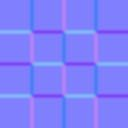# Modify Normal node

Left Toolbar >Normal Nodes >Modify Normal

The Modify Normal node modifies the normals of an image in Normal Color Mode.

inputoutputThe X and Y components of the normals have been inverted, and the strength of the normal has been multiplied by 3.0.

## Procedure

### To modify the normals of an image in Normal Color Mode:

1. On the left toolbar choose Normal Nodes > Modify Normal.>2. Drag the Modify Normal icon into the Node Graph.3. Connect the output of an image node into the Img input in the Modify Normal node.

4. Adjust the attributes of the Modify Normal node to modify the output normal image.

## Inputs

Img
An image in Normal Color Mode.

An image in Color Color Mode that will be used for masking zones in the input image and preventing them to get modified.

## Outputs

Img
An image in Normal Color Mode with the modifications applied.

## Attributes PanelInvert X
Inverts the X channel of the output normal.

Invert Y
Inverts the Y channel of the output normal.

Invert Z
Inverts the Z channel of the output normal.

Swap XZ
Swaps the X and Z component of the output normal.

Multiply
Multiplies the output angle by the given value, increasing or decreasing the strenght of the normal map.